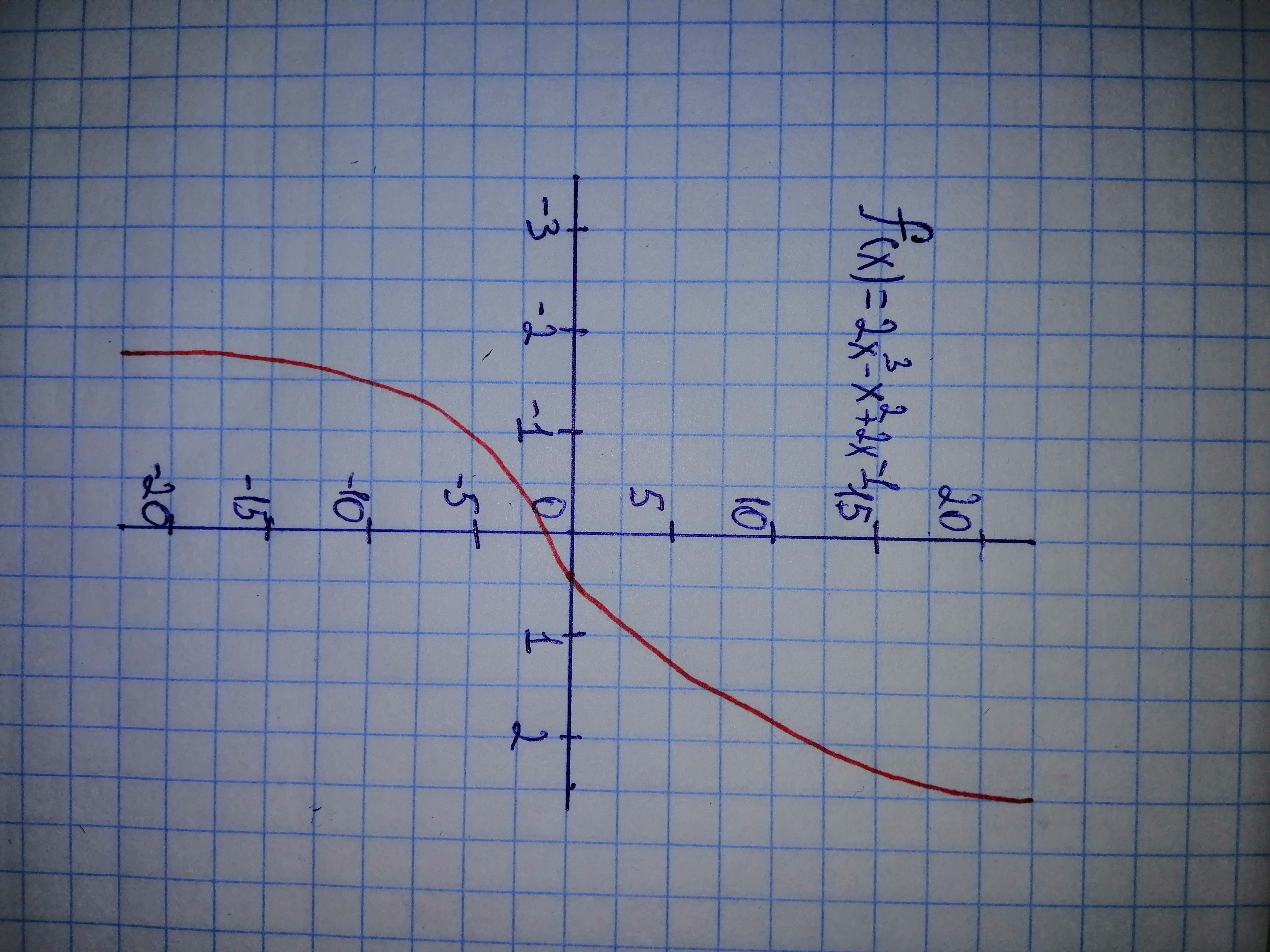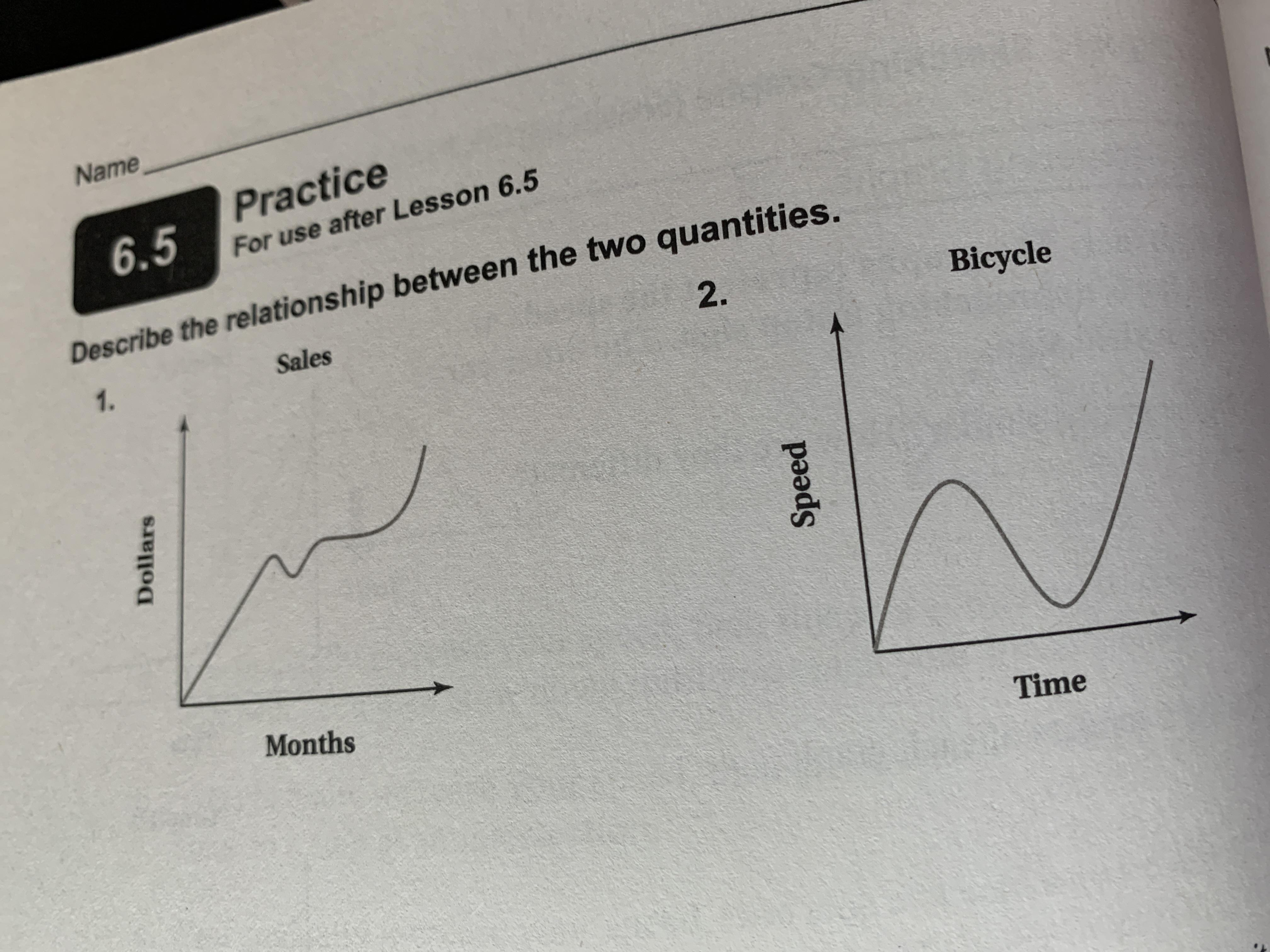Ask question

# Graph each polynomial function.f(x)=2x^{3} - x^{2} + 2x - 1 PSZ# Graph each polynomial function.f(x)=2x^{3} - x^{2} + 2x - 1 PSZ

Question
Polynomial graphsasked 2021-02-03

Graph each polynomial function. $$f(x)=2x^{3}\ -\ x^{2}\ +\ 2x\ -\ 1$$

## Answers (1)2021-02-04### Relevant Questionsasked 2021-02-02

(a) find the Maclaurin polynomial $$\displaystyle{P}_{{{3}}}{\left({x}\right)}$$ for PSKf(x), (b) complete the following

$$\begin{array}{|c|c|}\hline x: & -0.75 & -0.50 & -0.25 & 0 & 0.25 & 0.50 & 0.75 \\ \hline \end{array}$$

for f(x) and $$P_{3}(x)$$ and (c) sketch the graphs of f(x) and $$\displaystyle{P}_{{{3}}}{\left({x}\right)}$$ on the same set of coordinate axes. $$\displaystyle{f{{\left({x}\right)}}}={\arctan{{x}}}$$asked 2020-11-08

Graph each polynomial function. Factor first if the expression is not in factored form. $$f(x)=x^{3}\ +\ 3x^{2}\ -\ 13x\ -\ 15$$asked 2021-01-31

Simplify each expression.
1) $$-n+9n+3-8-8n$$
2) $$3(-4x+5y)-3x(2+4)$$
3) $$5-4y+x+9y$$
4) $$-2x+3y-5x-(-8y)$$asked 2021-02-08

Nested Form of a Polynomial Expand Q to prove that the polynomials P and Q ae the same $$\displaystyle{P}{\left({x}\right)}={3}{x}^{{4}}-{5}{x}^{{3}}+{x}^{{2}}-{3}{x}+{5}\ {Q}{\left({x}\right)}={\left({\left({\left({3}{x}-{5}\right)}{x}+{1}\right)}{x}-{3}\right)}{x}+{5}$$ Try to evaluate P(2) and Q(2) in your head, using the forms given. Which is easier? Now write the polynomial $$\displaystyle{R}{\left({x}\right)}={x}^{{5}}—{2}{x}^{{4}}+{3}{x}^{{3}}—{2}{x}^{{3}}+{3}{x}+{4}$$ in “nested” form, like the polynomial Q. Use the nested form to find R(3) in your head. Do you see how calculating with the nested form follows the same arithmetic steps as calculating the value ofa polynomial using synthetic division?asked 2020-12-25

Polynomial equation with real coefficients that has the roots $$3, 1 -i\ \text{is}\ x^{3} - 5x^{2} + 8x - 6 = 0.$$asked 2021-02-09

What is the process to solve these:
Vertex, $$y-\int$$., $$x-\int$$, graph $$= -(x+1)^2+1$$asked 2021-03-18

Describe the relationship between the two quantities.asked 2020-12-03

A graph of a linear equation passes through ( -2,0) and (0,-6) is the $$3x-y=6$$, both ordered pairs solutions for the equationasked 2021-01-02

Solve linear equation and check: $$7x+3=6(x-1)+9$$asked 2020-10-26

Determine the value of k for which the point (- 2, 3) lies on the line whose equation is .

$$4x+3ky=10$$

...